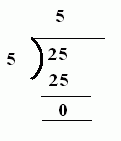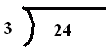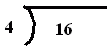Name: ___________________Date:___________________

 Email us to get an instant 20% discount on highly effective K-12 Math & English kwizNET Programs!

### Grade 4 - Mathematics5.4 Division using '')''

 Division can be written horizontally as 25/5 or 25 ÷ 5 Division problem may be written vertically as 5)25(Method: Divide the dividend by the divisor. The result is the quotient. Answer: 5 Directions: Divide the following. Also write at least 10 examples of your own.
 Q 1: Solve the following division problem.6598 Q 2: Solve the following division problem.6897 Q 3: Solve the following division problem.9687 Q 4: Solve the following division problem.8465 Q 5: Solve the following division problem.9867 Q 6: Solve the following division problem.6348 Q 7: Solve the following division problem.903910 Q 8: Solve the following division problem.13121910 Question 9: This question is available to subscribers only! Question 10: This question is available to subscribers only!

#### Subscription to kwizNET Learning System costs less than \$1 per month & offers the following benefits:

• Unrestricted access to grade appropriate lessons, quizzes, & printable worksheets
• Instant scoring of online quizzes
• Progress tracking and award certificates to keep your student motivated
• Unlimited practice with auto-generated 'WIZ MATH' quizzes